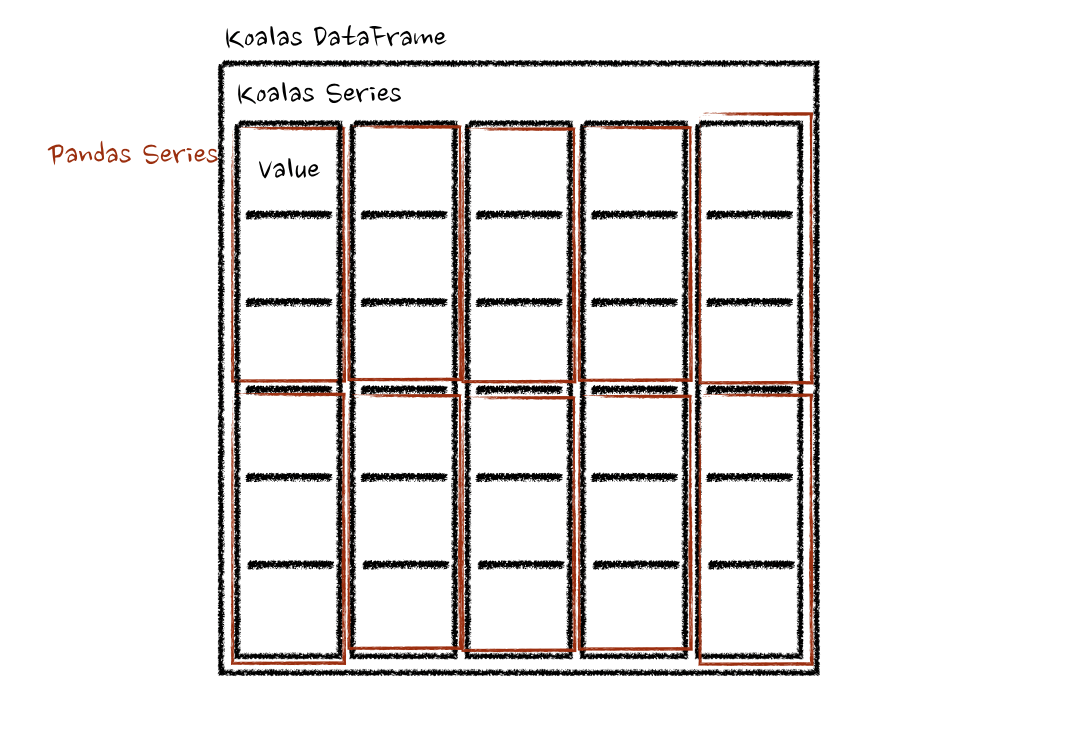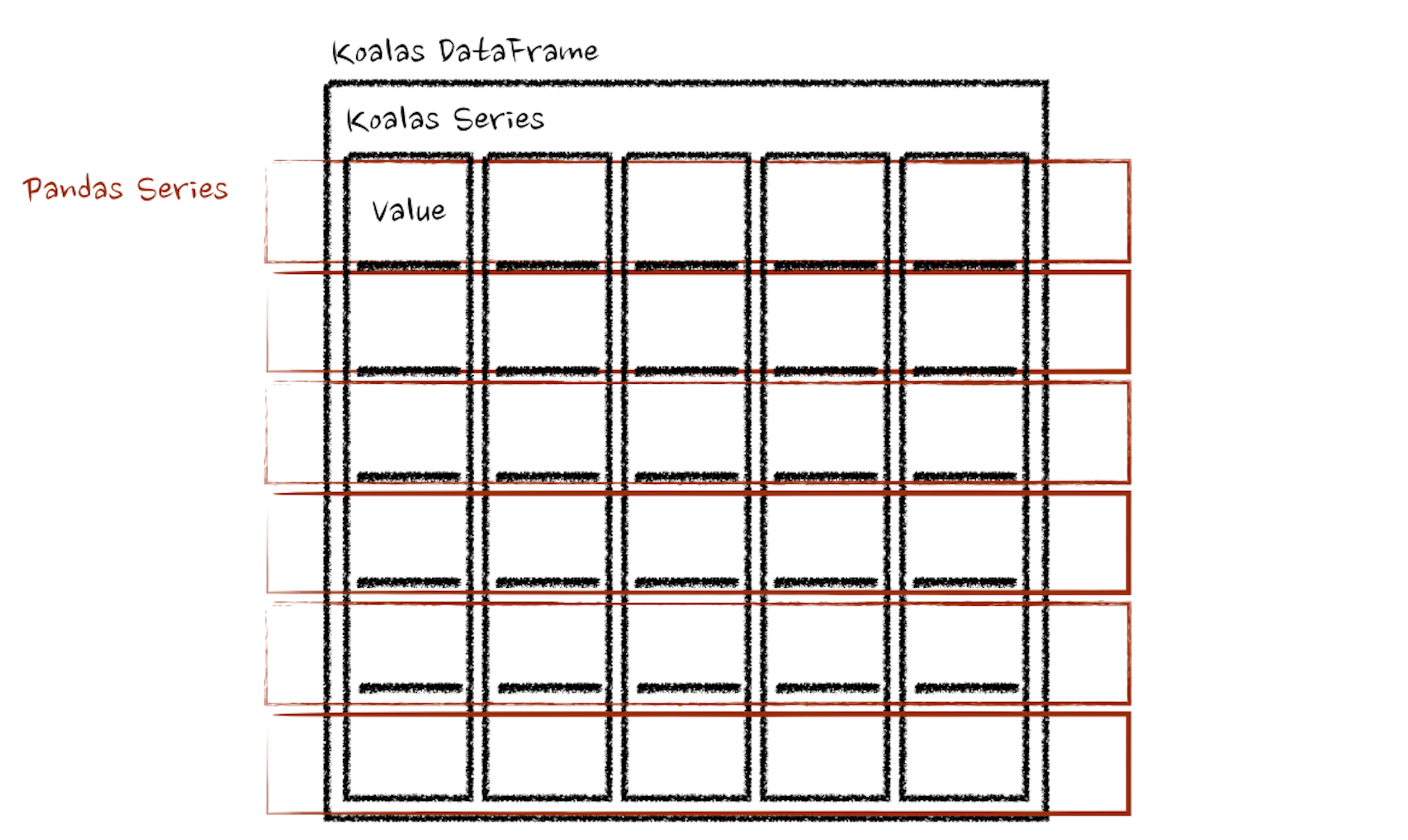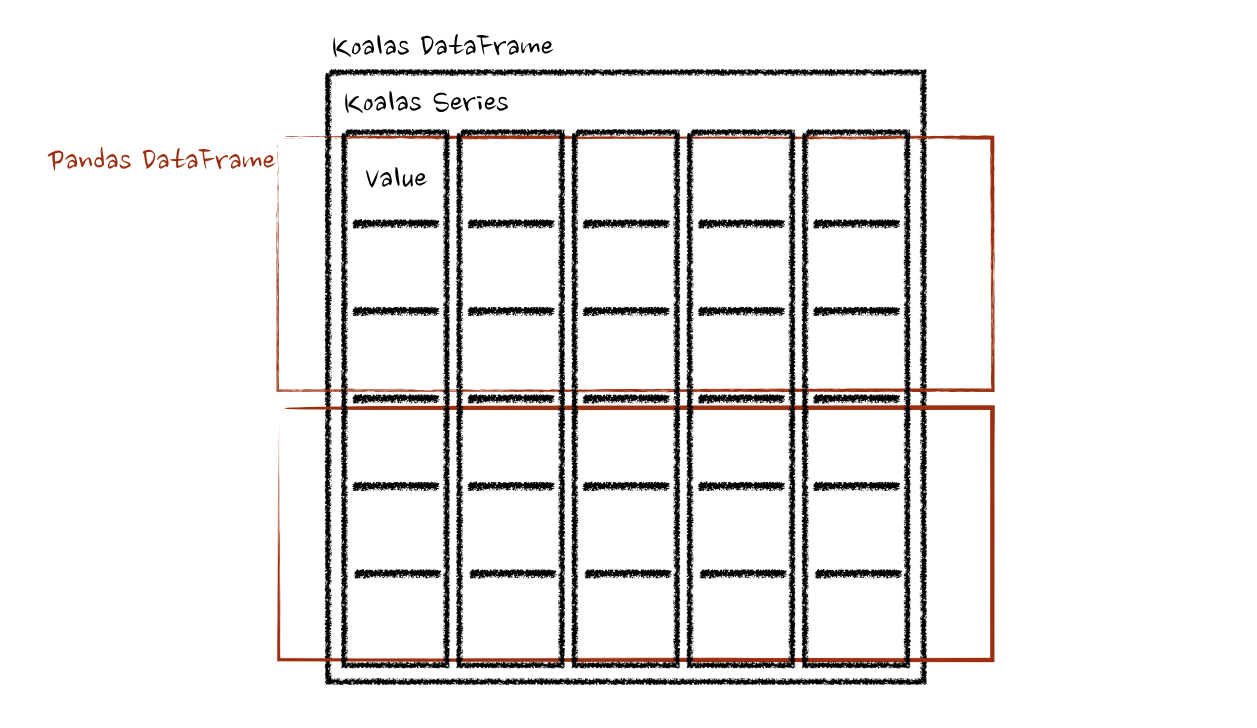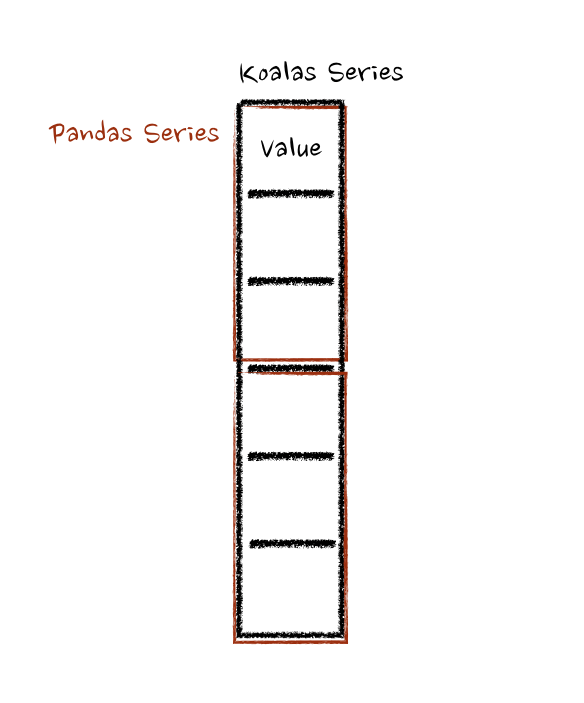# Transform and apply a function¶

There are many APIs that allow users to apply a function against Koalas DataFrame such as DataFrame.transform(), DataFrame.apply(), DataFrame.koalas.transform_batch(), DataFrame.koalas.apply_batch(), Series.koalas.transform_batch(), etc. Each has a distinct purpose and works differently internally. This section describes the differences among them where users are confused often.

## transform and apply¶

The main difference between DataFrame.transform() and DataFrame.apply() is that the former requires to return the same length of the input and the latter does not require this. See the example below:

>>> kdf = ks.DataFrame({'a': [1,2,3], 'b':[4,5,6]})
>>> def pandas_plus(pser):
...     return pser + 1  # should always return the same length as input.
...
>>> kdf.transform(pandas_plus)

>>> kdf = ks.DataFrame({'a': [1,2,3], 'b':[5,6,7]})
>>> def pandas_plus(pser):
...     return pser[pser % 2 == 1]  # allows an arbitrary length
...
>>> kdf.apply(pandas_plus)


In this case, each function takes a pandas Series, and Koalas computes the functions in a distributed manner as below.In case of ‘column’ axis, the function takes each row as a pandas Series.

>>> kdf = ks.DataFrame({'a': [1,2,3], 'b':[4,5,6]})
>>> def pandas_plus(pser):
...     return sum(pser)  # allows an arbitrary length
...
>>> kdf.apply(pandas_plus, axis='columns')


The example above calculates the summation of each row as a pandas Series. See below:In the examples above, the type hints were not used for simplicity but it is encouraged to use to avoid performance penalty. Please refer the API documentations.

## koalas.transform_batch and koalas.apply_batch¶

In DataFrame.koalas.transform_batch(), DataFrame.koalas.apply_batch(), Series.koalas.transform_batch(), etc., the batch postfix means each chunk in Koalas DataFrame or Series. The APIs slice the Koalas DataFrame or Series, and then applies the given function with pandas DataFrame or Series as input and output. See the examples below:

>>> kdf = ks.DataFrame({'a': [1,2,3], 'b':[4,5,6]})
>>> def pandas_plus(pdf):
...     return pdf + 1  # should always return the same length as input.
...
>>> kdf.koalas.transform_batch(pandas_plus)

>>> kdf = ks.DataFrame({'a': [1,2,3], 'b':[4,5,6]})
>>> def pandas_plus(pdf):
...     return pdf[pdf.a > 1]  # allow arbitrary length
...
>>> kdf.koalas.apply_batch(pandas_plus)


The functions in both examples take a pandas DataFrame as a chunk of Koalas DataFrame, and output a pandas DataFrame. Koalas combines the pandas DataFrames as a Koalas DataFrame.

Note that DataFrame.koalas.transform_batch() has the length restriction - the length of input and output should be the same whereas DataFrame.koalas.apply_batch() does not. However, it is important to know that the output belongs to the same DataFrame when DataFrame.koalas.transform_batch() returns a Series, and you can avoid a shuffle by the operations between different DataFrames. In case of DataFrame.koalas.apply_batch(), its output is always treated that it belongs to a new different DataFrame. See also Operations on different DataFrames for more details.In case of Series.koalas.transform_batch(), it is also similar with DataFrame.koalas.transform_batch(); however, it takes a pandas Series as a chunk of Koalas Series.

>>> kdf = ks.DataFrame({'a': [1,2,3], 'b':[4,5,6]})
>>> def pandas_plus(pser):
...     return pser + 1  # should always return the same length as input.
...
>>> kdf.a.koalas.transform_batch(pandas_plus)


Under the hood, each batch of Koalas Series is split to multiple pandas Series, and each function computes on that as below:There are more details such as the type inference and preventing its performance penalty. Please refer the API documentations.